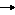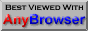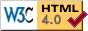## Chapter 5 - Numeric Data Types

As we have noted above, there are three types of data that are considered numeric, these are:

Bytes

The range of a byte is 0 to 255. Whole numbers only, no decimal places, please. It can be used to represent the ASCII code for single characters or for conserving memory if you are sure the range is large enough for your data.

Integers

An integer, like a byte, can only contain a whole number. Unlike a byte, it can be negative and the range can be much greater, as seen from the table in the previous chapter. It is advisable to use them wherever possible because access and manipulation is fastest.

Floating point

Floating point numbers, or real numbers as some refer to them, are numbers that contain decimal places. If you were representing the square root of 2 (1.4142) or acceleration due to gravity (9.81) you would use a floating point number. Floating point numbers are also used to represent very big numbers and very small numbers. Examples of these would be things like the speed of light (3.0E8) or the distances between atomic particles (1.0E-10). The 'E' must always be uppercase, unless you override the defaults.

With the noted restriction on ranges, you can move freely between numeric types, BASIC will take care of the details for you, for example if you have a floating point number, say, 3.14159 and you assign it to an integer variable, BASIC will chop off the .14159 and you are left with 3. In other languages, you have to supply a specific conversion, or you get an error. BASIC will do this without prompting which can be a blessing or a curse, depending on how you look at it.

We have already dealt with the four basic mathematical operators: + – * /. What else can we do with numbers? Well, lots, actually. For a start, there are three additional operations that I would like to introduce.

To try most of these examples, you can use immediate mode and type them straight in. To select immediate mode, go to the toolbar and click the button with the picture of a keyboard which is second to the end, next to help. An output window will open and you can type the commands in without having to keep closing the window and edit the code. There are some programs in here too, if it's coloured, you need the editor; if it's black, the editor or immediate mode.

Powers of numbers ^

Using ^, we can raise a number to the power of another number:

 PRINT 4^2
Which is four squared. Or:
 PRINT 4^0.5
Which is the square root of 4: 2. The power can be pretty much any number or numeric variable you like, just be sure that the recipient variable is of the correct type to handle the result.

MOD and DIV

MOD gives us the remainder after a division.

 PRINT 20 MOD 7
The answer is 6. 7 * 2 = 14 and 20 – 14 leaves us with 6. To see if a number is odd or even we could MOD it by 2. If the result is 0, 2 went into it with no remainder: it's even. If the result is 1, it must be odd. Try this several times with different numbers:
 PRINT 43 MOD 2 PRINT 666 MOD 2
DIV gives us the number of times the divisor went into the dividend and throws away the remainder (if any).
 PRINT 20 DIV 7
We get 2. These two functions have many uses, for example working out where to print a character on a screen, which should be a whole number.

Variables that change themselves

Look at this line:

 I%=I%+20
Anyone from a strict mathematical background might think this doesn't make sense. How can I% be equal to I% plus 20. In a computer language, this is perfectly legal. When BASIC does a calculation, it uses a scratchpad area. What it does is get the current value from I%, put it in this working area and add 20 to it. Then it takes the result and puts it back in the variable location called I%.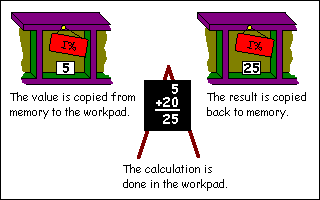You'll see this lots, in fact it's so common that BBC BASIC has a shorthand way of writing it. I could try and explain it but an example is far easier, the above would become:

 I%+=20
It does the same job as the previous line, just saves typing and bytes for the demo version users. If you need to verbalise this when you see it try saying something like: "I% is increased by 20". You can do this for all the mathematical operations mentioned above, with the exception of powers. Here is an entirely meaningless program that demonstrates their usage.
 I%+=1   : REM I% increases by 1 I%-=1   : REM I% decreased by 1 I%*=10  : REM I% multiplied by 10 I%/=2   : REM I% divided by 2 I%MOD=2 : REM I% MODed by 2 I%DIV=2 : REM I% DIVed (!) by 2 END
Spaces are not important here. The lines could be written as:
 I% + = 20
However, I prefer to keep the operation and the equals sign together as it's easier to read.

Mathematical Functions

As well as the operations described above, we have an entire armoury of functions that allow us to manipulate numerical values. A function, in this case, is a keyword that can be used in a mathematical expression as it returns a value. Functions usually have a value passed to them which they do something with and return the result. The value passed is called the parameter or argument.

The help files will tell you that brackets are not always necessary when calling functions, but include them as it makes the program easier to understand and it's less to remember in the early days. So, without further ado, here are the mathematical functions that BBC BASIC supports.

Standard Functions

ABS(X)

ABS returns the absolute value of a number. Simply put if X is negative, ABS(X) gives us the positive value.

 PRINT ABS(-5.5) PRINT ABS(6.6)
Give 5.5 and 6.6 respectively. ABS can be used to find the difference between two numbers without needing to decide which is the higher value:
 REM ABS demo A=32.5 B=43.6 PRINT ABS(A-B) END
SGN(X)

SGN stands for sign or signum if you don't want to get confused with sine. If X is negative, it returns –1. If X is zero, it returns 0 and if it's positive, you get +1. Try these:

 PRINT SGN(23) PRINT SGN(-5) PRINT SGN(3-3) PRINT SGN(25-(2*23))
INT(X)

INT rounds a number down to the nearest integer value. For positive numbers, this is pretty obvious:

 PRINT INT(3.14159) PRINT INT(99.99)
For negative ones, remember that rounding down might give unexpected (though completely explainable) results:
 PRINT INT(-3.14159) PRINT INT(-99.99)
Tip: Rounding to the nearest integer

If you want to round a number to the nearest whole integer, add 0.5 to it first. This way, if the number is between .0 to .4, it is rounded down and if it is between .5 and .9, it is rounded up.

 REM Rounding with INT A=3.14159 B=99.99 PRINT INT(A+0.5) PRINT INT(B+0.5) END

LN(X) and EXP(X)

LN returns the natural logarithm (base 'e' where e = 2.7183...) of X. EXP returns 'e' raised to the power of X.

 REM LN and EXP A=3.4 B=LN(A) C=EXP(B) PRINT A;",";B;",";C END
LOG(X)

LOG returns the logarithm to base 10 of X. There is no opposite antilog function, like LN/EXP. This is quite easy to find however, all we do is raise 10 to the power of the number we wish to antilog:

 REM LOG and antilog A=3.4 B=LOG(A) C=10^B PRINT A;",";B;",";C END
SQR(X)

It is possible to find any root of a number by using X^(1/RootRequired). As finding the square root of a number is such a common operation, this keyword is provided for that purpose. Now you can do Pythagoras until the cows come home:

 REM SQR and right angled triangles Side1=3 Side2=4 Hyp=SQR(Side1^2 + Side2^2) PRINT Hyp END
Trigonometric functions

PI

The online help says this is a function. In actual usage, it takes no parameters, so you just use it like a variable that you can't change. It returns a value for good old 22/7 (approximately), used in lots of calculations for circles and angles.

All the trigonometric functions work with radians. There are PI radians in 180 degrees (half a circle), so 1 degree = PI/180 radians. To make life a lot more tolerable, DEG(X) will convert a value in radians, X, into degrees and ... wait for it ... RAD(X) converts a value in degrees into radians.

SIN(X), COS(X) and TAN(X)

These supply the sine, cosine and tangent of a given angle X. As noted above, all these work in radians, but conversion is painless thanks to DEG and RAD.

ACS(X), ASN(X) and ATN(X)

Given a value X, these will return the corresponding angle in radians for arcsine, arccosine and arctangent.

Special functions

RND(X)

This is the game programmer's favourite function. It returns a random number, great for deciding when aliens should swoop down from the sky, or how much strength you need to wield the Club of Maiming or ... Actually, it's quite a complex beast depending on the value you pass as X. Let's examine the options.

(a) RND with no argument returns a random integer in the range –2147483648 to +2147483647, which is the highest value a 32 bit signed number can hold.

 PRINT RND
(b) RND(X%) where X% is a positive integer value greater than 1. This will return a random number in the range 1 up to and including X%
 PRINT RND(10)
Tip: Generating a random number for different ranges
If you need to generate a random number between, say, 6 and 15, you do this by using RND(10) to get a number and adding an offset to it.

 PRINT RND(10)+5

(c) RND(1) returns a floating point number in the range 0.0 up to but not including 1.0
 PRINT RND(1)
Tip: Converting RND
If converting other dialects of BASIC, this is the usual one that is supplied. To get an integer value you can do this:

 PRINT INT(RND(1)*10+1)
or you can use RND(X%) as described previously.

(d) RND(0) will return the last random number again, but as a floating point number as described in (c).

(e) RND(X) where X is a negative number. This will seed the random number generator to start from a new number. The numbers generated by RND are not truly random, but there are so many that it would take you a long time to detect when they started to repeat. If you were really bored, you could generate them and find the pattern. Although the random number sequence is set each time BBC BASIC starts so that it doesn't produce the same number, sometimes it is necessary to make the random number generator start from a predefined place in its sequence, this is where the negative number is used.

Operator Precedence

Let's go into immediate mode and try an experiment. Try and predict the result of this line before pressing return.

 PRINT 2+3*4
OK, what did you get? Was it the answer you expected? If you predicted 14, you are already familiar with operator precedence. If you expected 20, you might be a bit puzzled, so stick with it and I'll explain. Operator precedence is another phrase for the priority of a mathematical operation. In common maths, multiplication and division have a higher priority than addition and subtraction. Applying this knowledge, we can now break down how our beloved BASIC arrived at the result 14. When presented with the expression, something like this happens:
 2+3*4 Multiplication found, do this first 2+12 Now do the addition = 14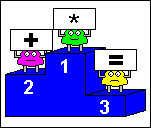If you want to force BASIC to override the priority, you need to use parentheses:

 PRINT (2+3)*4
This will give 20 as the expression in brackets is forced to be done first. Any calculations within the parenthesis would be done according to the previous rules, including any further expressions with brackets.

Slowly we could build up a list of operation against priority, but to save time, here is the complete list:

 Priority Operation Highest literal values, variables, functions, parentheses (), unary+–, NOT power ^ multiplication *, division /,  MOD,  DIV addition +, subtraction – comparisons =  <>  <=  >=  >  < , shifts <<  >>  >>> AND EOR, OR Lowest assignment =

Some of these won't make sense yet, such as the comparison operations, but rather than keep updating the list as we go through, it's all here for you to refer back to. Notice that the lowest priority is the assignment instruction. This means that absolutely everything is done before the value is assigned to the variable lined up to receive the result, which is what you'd expect isn't it?

Exercises

1) Modify the circle program to use PI instead of 3.14159. Save it.
2) Write a program that uses RND to simulate two six-sided dice being thrown. Print the results for each die and the total.
3) Verify that the following formula is true:

 LOG(X)=LN(X)/LN(10)

### CHAPTER 6